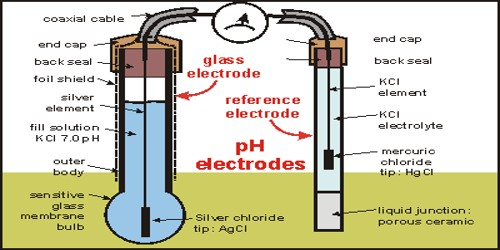# Glass Electrode: the pH Meter

Glass electrode: the pH meter

At the present time in most applications pH is measured with the help of a glass electrode coupled with a standard calomel electrode.

A glass electrode (Figure) consists of a silver wire coated with silver chloride immersed in a solution of hydrochloric acid solution of constant pH (buffer solution) and placed inside a container made of special glass. The potential of the glass electrode is found to change with the hydrogen ion concentration of the solution in which it is immersed, i.e. it is reversible with respect to the H+ ions. Using Nernst equation for the electrode reaction at the glass electrode-

2H+ (aq) + 2e → H2 (g)

the potential of this glass electrode is given by

Eglass = E0glass – (0.0591/2) log [H2(g)] / [H+]2

= E0glass + (0.0591) log [H+]

= E0glass – (- 0.0591) log [H+]

= E0glass – 0.0591 pH … … … (1)In order to determine the pH of an unknown solution the glass electrode and the calomel electrode both are dipped in the solution up the following cell.

Glass electrode│Solution of unknown pH│ Calomel electrode

The e.m.f. of the cell is given by-

Ecell = Ecalomel – Eglass … … … (2)

Substituting Equation (1) and (2) we get,

Ecell = Ecalomel – (E0glass – 0.0591 pH)

So, pH = 9Ecell – Ecalomel + Eglass) / 0.0591

Thus the pH of an unknown solution can be easily determined by measuring the e.m.f. of the cell, since Ecalomel and Eglass (Eglass can be determined by using a solution of known pH in the above cell) are known. In fact in commercial pH meters the potential values are convened into pH values which are directly read from the dial of the instrument.

Glass electrodes arc commercially available as these are simple to use and are not affected by oxidizing and reducing agents.